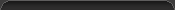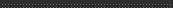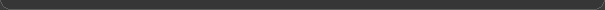DocumentationBasic Introduction    FAQ    Troubleshooting    How WhiteCap Works    License Agreement    ContactAdvanced Standalone Use    Customizing    Scripting    Config Programming    Version HistoryConfig Programming
G-Force and WhiteCap use plain-text files called "configs" in order to define graphical operations in the form of mathematical expressions, allowing you to create your own visual effects. Over the years, many users have created hundreds of configs, some of which are now bundled with G-Force. A config file is a plain-text file and contains a list of values than can be easily viewed or edited using any text editor. Depending on the role a config file is intended to play, G-Force looks for specifically named values and uses them in accordance with the role of that config. For example, when a WaveShape config defines a value named "Pen", that value determines how darkly lines are drawn.

If you'd like your configs to be bundled with G-Force, you should submit them. When you submit configs to SoundSpectrum, they will be evaluated and considered for the next release of G-Force. Even if your configs are not included in the next release, your work will be posted on the G-Force contributors page and will be available to all. By creating configs and sharing them, you'll be making the G-Force community even more exciting and you'll be appreciated by everyone who uses G-Force. Finally, G-Force config contributors are eligible for a free copy of G-Force Platinum (see the G-Force contributors page for more information).

Syntax

The config file syntax is straightforward and is nothing more than a unordered list of key-value pairs. Configs are plain-text files that are edited using a text editor (see the comments in the customizing section regarding text editors). Each line in a config file starts with a 1 to 4 character case-sensitive label or "key" followed by an equal sign, followed by the key's value. If the value isn't a number (with the exception of a leading '-' or '.'), then it should be in quotes. All whitespace or new lines not inside quotes are ignored, and both types of C-style comments are processed. An example:
X0=".5 + sin( .3 * t )"    // This is a comment
Y0=".1234"
Pen=.8
Ans=-42
ALFA="1 / exp( C1 ^ 2 )"/*     This is another comment */
Vers=310

Documented Configs

Below is a list of configs included with G-Force that contain important documentation. In other words, some configs contain information that will teach you about the different config types. Try looking at them in order listed, and be aware that many other configs contain documentation and comments added by the authors who created them. If you see a config in G-Force you really like, don't be afraid to open and study it.
1. G-Force/ColorMaps/ColorWheel.txt
2. G-Force/ColorMaps/Clear Blue Water.txt
4. G-Force/WaveShapes/Rotating Corridor.txt
5. G-Force/Particles/Spinners.txt
6. G-Force/Particles (Non-Auto)/Default Sprite.txt
7. G-Force/FlowField Unions/Birth Cone.txt

Be Patient

Don't get discouraged if the math seems a little overwhelming. It's simply a matter of experience and it will become easier with just a little time and practice. As you explore existing configs, you may want to try using a graphing calculator to help see how certain equations behave. A common way to learn config programming is to alter existing configs and observe the effects of your changes.

When you're ready to test your config, you have to tell G-Force to load a fresh copy of it from disk. Otherwise, if you switch to a config you've been editing, G-Force won't load your changes (because it's using a cached copy). Press U to reload the currently running configs. In other words, when you save changes to a config, press U to make G-Force load a fresh copy from disk.

Config/Language Reference

Common

 abs(x) |x| dim(a,b) max( a - b, 0 ) sqr(x) x^2 exp(x) e^x log(x) natural logarithm (logarithm base e) log10(x) logarithm base 10 sqrt(x) square root of x sin(x) sine (x is in radians) cos(x) cosine (x is in radians) tan(x) tangent (x is in radians) asin(x) arcsine (range is -PI/2 to PI/2) acos(x) arccosine (range is 0 to PI) atan(x) arctangent (range is -PI/2 to PI/2) atan2(y,x) arctangent of y/x (range is -PI to PI) hypot(x,y) sqrt( x^2 + y^2 ) hypot2(x,y) x^2 + y^2 leg(h,x) sqrt( max( h^2 - x^2, 0 ) ) wyvill(r) -4/9 r^3 + 17/9 r^2 - 22/9 r + 1

Logical/Discrete

 pos(x) returns x if x > 0 and 0 otherwise sign(x) returns 1 if x >= 0 and -1 otherwise clamp(x) returns 0 when x < 0, 1 when x > 1, and x when 0 <= x <= 1 sqwv(x) returns 1 if |x| <= 1.0 and 0 otherwise

Miscellaneous

 rnd(x) returns a random real number from 0 to x (inclusive) trunc(x) everything to the right of the decimal point in x is dropped floor(x) the largest integer that is less than or equal to x (ex, floor(3.2) == 3, floor(-2.7) == -3 ) round(x) returns the whole number nearest to x wrap(x) x - floor( x ) (ex: wrap(.3) == .3, wrap(4.1) == .1, wrap(-2.7) == .3) flip(x) sawtooth that passes thru ..., (-3, -1), (-1, 1), (-1, -1), (1,1), (1, -1), ... trwv(x) triangle-shaped wave passing through (0,0), (1,1), (2,0) and repeats in both directions along the x axis min(a,b) if a <= b, returns a, otherwise returns b max(a,b) if a >= b, returns a, otherwise returns b srand(x) seeds the random number generator based on the bits of x, returns x clock() returns the system time index (in milliseconds) time() returns the number of seconds elapsed since midnight

Operators

 +, - add/subtract *, / multiply/divide % modulo or "the integer remainder when A is divided by B" (ex, 8 % 4 == 0, 11 % 3 == 2) ^ raise to the power ==, != equal/unequal to <, > less/greater than <=, >= less/greater than or equal to && logical AND || logical OR & bitwise AND | bitwise OR ^^ bitwise XOR <<, >> shift left/right (int) convert to type int (unary operator) (float) convert to type float (unary operator)

Audio Access

 mag(x) Accesses samples of the current audio fft(x) Accesses the frequency transform/spectrum of the current audio BASS A single scalar value that approximates the overall beat

Globals

 PI 3.141592653 NUM_S_STEPS The number of times the X, Y, and C expressions are evaluated per sample (ie, per frame) NUM_FFT_BINS The number of bins/resolution composing fft() NUM_SAMPLE_BINS The number of values composing mag() HEIGHT, WIDTH The output frame dimensions (in pixels). Because the frame size can change at any time, these globals should be treated as time dependent when used inside a WaveShape config. X_EXTENTS, Y_EXTENTS The four points (+/- X _EXTENTS, +/- Y_EXTENTS) always map to the corners of the output frame. Because the frame size can change at any time, these globals should be treated as time dependent when used inside a WaveShape config. When a FlowField or WaveShape does not have a forced 1:1 aspect (ie, if Aspc=0), then both these globals are therefore 1.

Deprecated

 sgn(x) use sign(x) atan2(x) use atan2(y,x) flor(x) use floor(x) trnc(x) use trunc(x) clip(x) use clamp(x) seed(x) use srand(x)